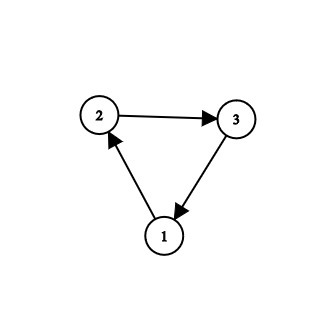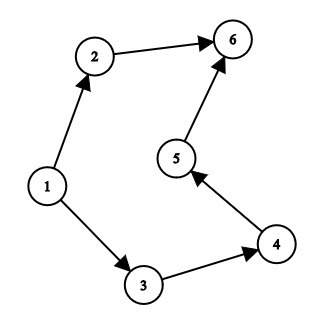# Find dependencies of each Vertex in a Directed Graph

• Difficulty Level : Hard
• Last Updated : 09 Feb, 2021

Given a directed graph containing N vertices and M edges, the task is to find all the dependencies of each vertex in the graph and the vertex with the minimum dependency.

A directed graph (or digraph) is a set of nodes connected by edges, where the edges have a direction associated with them.
For example, an arc (x, y) is considered to be directed from x to y, and the arc (y, x) is the inverted link. Y is a direct successor of x, and x is a direct predecessor of y.
The dependency is the number of connections to different vertices which are dependent on the current vertex.

Examples:

Input:Output:
Vertex 1 dependencies -> 2-> 3
Vertex 2 dependencies -> 3-> 1
Vertex 3 dependencies -> 1-> 2
Node 1 has the minimum number of dependency of 2.
Explanation:
Vertex 1 is dependent on 2 and 3.
Similarly, vertex 2 and 3 on (3, 1) and (1, 2) respectively.
Therefore, the minimum number of dependency among all vertices is 2.
Input:Output:
Vertex 1 dependency -> 2-> 3-> 4-> 5-> 6
Vertex 2 dependency -> 6
Vertex 3 dependency -> 4-> 5-> 6
Vertex 4 dependency -> 5-> 6
Vertex 5 dependency -> 6
Vertex 6 is not dependent on any vertex.
Node 6 has the minimum dependency of 0
Explanation:
Vertex 1 is dependent on (3, 4, 5, 6, 7). Similarly, vertex 2 on (6), vertex 3 on (4, 5, 6), vertex 4 on (5, 6), vertex 5 on (6) and vertex 6 is not dependent on any.
Therefore, the minimum number of dependency among all vertices is 0.

Approach: The idea is to use depth-first search(DFS) to solve this problem.

• Get the directed graph as the input.
• Perform the DFS on the graph and explore all the nodes of the graph.
• While exploring the neighbours of the node, add 1 to count and finally return the count which signifies the number of dependencies.
• Finally, find the node with the minimum number of dependencies.

Below is the implementation of the above approach:

## CPP

 `// C++ program to find the``// dependency of each node` `#include ``using` `namespace` `std;` `// Defining the graph``class` `Graph {` `    ``// Variable to store the``    ``// number of vertices``    ``int` `V;` `    ``// Adjacency list``    ``list<``int``>* adjList;` `    ``// Initializing the graph``public``:``    ``Graph(``int` `v)``    ``{``        ``V = v;``        ``adjList = ``new` `list<``int``>[V];``    ``}` `    ``// Adding edges``    ``void` `addEdge(``int` `u, ``int` `v,``                 ``bool` `bidir = ``true``)``    ``{``        ``adjList[u].push_back(v);``        ``if` `(bidir) {``            ``adjList[u].push_back(v);``        ``}``    ``}` `    ``// Performing DFS on each node``    ``int` `dfs(``int` `src)``    ``{``        ``// Map is used to mark``        ``// the current node as visited``        ``map<``int``, ``bool``> visited;``        ``vector<``int``> dependent;``        ``int` `count = 0;` `        ``stack<``int``> s;` `        ``// Push the current vertex``        ``// to the stack which``        ``// stores the result``        ``s.push(src);` `        ``visited[src] = ``true``;` `        ``// Traverse through the vertices``        ``// until the stack is empty``        ``while` `(!s.empty()) {``            ``int` `n = s.top();``            ``s.pop();` `            ``// Recur for all the vertices``            ``// adjacent to this vertex``            ``for` `(``auto` `i : adjList[n]) {` `                ``// If the vertices are``                ``// not visited``                ``if` `(!visited[i]) {``                    ``dependent.push_back(i + 1);``                    ``count++;` `                    ``// Mark the vertex as``                    ``// visited``                    ``visited[i] = ``true``;` `                    ``// Push the current vertex to``                    ``// the stack which stores``                    ``// the result``                    ``s.push(i);``                ``}``            ``}``        ``}` `        ``// If the vertex has 0 dependency``        ``if` `(!count) {``            ``cout << ``"Vertex "` `<< src + 1``                 ``<< ``" is not dependent on any vertex.\n"``;``            ``return` `count;``        ``}` `        ``cout << ``"Vertex "` `<< src + 1 << ``" dependency "``;``        ``for` `(``auto` `i : dependent) {``            ``cout << ``"-> "` `<< i;``        ``}``        ``cout << ``"\n"``;``        ``return` `count;``    ``}``};` `// Function to find the``// dependency of each node``void` `operations(``int` `arr[],``                ``int` `n, ``int` `m)``{``    ``// Creating a new graph``    ``Graph g(n);` `    ``for` `(``int` `i = 0; i < m; i++) {``        ``g.addEdge(arr[i],``                  ``arr[i], ``false``);``    ``}` `    ``int` `ans = INT_MAX;``    ``int` `node = 0;` `    ``// Iterating through the graph``    ``for` `(``int` `i = 0; i < n; i++) {``        ``int` `c = g.dfs(i);` `        ``// Finding the node with``        ``// minimum number of``        ``// dependency``        ``if` `(c < ans) {``            ``ans = c;``            ``node = i + 1;``        ``}``    ``}``    ``cout << ``"Node "` `<< node``         ``<< ``"has minimum dependency of "``         ``<< ans;``}` `// Driver code``int` `main()``{``    ``int` `n, m;` `    ``n = 6, m = 6;` `    ``// Defining the edges of the``    ``// graph``    ``int` `arr[] = { { 0, 1 },``                     ``{ 0, 2 },``                     ``{ 2, 3 },``                     ``{ 4, 5 },``                     ``{ 3, 4 },``                     ``{ 1, 5 } };` `    ``operations(arr, n, m);` `    ``return` `0;``}`

## Python3

 `# Python3 program to find the``# dependency of each node` `# Adding edges``def` `addEdge(u, v, bidir ``=` `True``):``    ``global` `adjList``    ``adjList[u].append(v)``    ``if` `(bidir):``        ``adjList[u].append(v)` `# Performing DFS on each node``def` `dfs(src):``    ``global` `adjList, V``    ` `    ``# Map is used to mark``    ``# the current node as visited``    ``visited ``=` `[``False` `for` `i ``in` `range``(V``+``1``)]``    ``dependent ``=` `[]``    ``count ``=` `0``    ``s ``=` `[]` `    ``# Push the current vertex``    ``# to the stack which``    ``# stores the result``    ``s.append(src)``    ``visited[src] ``=` `True` `    ``# Traverse through the vertices``    ``# until the stack is empty``    ``while` `(``len``(s) > ``0``):``        ``n ``=` `s[``-``1``]``        ``del` `s[``-``1``]` `        ``# Recur for all the vertices``        ``# adjacent to this vertex``        ``for` `i ``in` `adjList[n]:` `            ``# If the vertices are``            ``# not visited``            ``if` `(``not` `visited[i]):``                ``dependent.append(i ``+` `1``)``                ``count ``+``=` `1` `                ``# Mark the vertex as``                ``# visited``                ``visited[i] ``=` `True` `                ``# Push the current vertex to``                ``# the stack which stores``                ``# the result``                ``s.append(i)` `    ``# If the vertex has 0 dependency``    ``if` `(``not` `count):``        ``print``(``"Vertex "``, src ``+` `1``,``              ``" is not dependent on any vertex."``)``        ``return` `count` `    ``print``(``"Vertex "``,src ``+` `1``,``" dependency "``,end``=``"")``    ``for` `i ``in` `dependent:``        ``print``(``"-> "``, i, end ``=` `"")``    ``print``()``    ``return` `count` `# Function to find the``# dependency of each node``def` `operations(arr, n, m):``  ` `    ``# Creating a new graph``    ``global` `adjList``    ``for` `i ``in` `range``(m):``        ``addEdge(arr[i][``0``], arr[i][``1``], ``False``)``    ``ans ``=` `10``*``*``18``    ``node ``=` `0` `    ``# Iterating through the graph``    ``for` `i ``in` `range``(n):``        ``c ``=` `dfs(i)` `        ``# Finding the node with``        ``# minimum number of``        ``# dependency``        ``if` `(c < ans):``            ``ans ``=` `c``            ``node ``=` `i ``+` `1``    ``print``(``"Node"``, node, ``"has minimum dependency of "``, ans)` `# Driver code``if` `__name__ ``=``=` `'__main__'``:``    ``V ``=` `6``    ``adjList ``=` `[[] ``for` `i ``in` `range``(V``+``1``)]``    ``n, m ``=` `6``, ``6`  `    ``# Defining the edges of the``    ``# graph``    ``arr ``=` `[ [ ``0``, ``1` `],``             ``[ ``0``, ``2` `],``             ``[ ``2``, ``3` `],``             ``[ ``4``, ``5` `],``             ``[ ``3``, ``4` `],``             ``[ ``1``, ``5` `] ]` `    ``operations(arr, n, m)` `    ``# This code is contributed by mohit kumar 29.`

Output:

```Vertex 1 dependency -> 2-> 3-> 4-> 5-> 6
Vertex 2 dependency -> 6
Vertex 3 dependency -> 4-> 5-> 6
Vertex 4 dependency -> 5-> 6
Vertex 5 dependency -> 6
Vertex 6 is not dependent on any vertex.
Node 6has minimum dependency of 0```

My Personal Notes arrow_drop_up